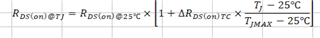If you have a related question, please click the "Ask a related question" button in the top right corner. The newly created question will be automatically linked to this question.

# CSD19501KCS: Clarification of a parameter in MOTOR-DRIVE-FET-LOSS-CALC

Part Number: CSD19501KCS
Other Parts Discussed in Thread: MOTOR-DRIVE-FET-LOSS-CALC,

Hi, In the MOTOR-DRIVE-FET-LOSS-CALC Excel sheet, the equations tab contains an equation to calculate RDS(on) with temperature. But I am unable to understand what ΔRDS(on)TC means. Is it the total change of RDson across the entire temp range?  I am attaching a screenshot below.• Hello Binil,

Thanks for your interest in TI FETs. Please refer to Figure 8 in the CSD19501KCS datasheet which is the normalized (= 1 at 25°C) on resistance of the FET vs.case temperature. The term ΔRDS(on)TC is the average slope of the curves assuming they are linear. The slope used for the CSD19501KCS is 0.94. If you plug 25°C into the equation, the multiplier is 1. If you plug 175°C into the equation, the multiplier is 1.94 which is actually a little low if you look at the graph. This is because I'm using a database from our Webench team that assumed TJmax is 150°C and this is not the case for the CSD19501KCS which is 175°C. It should be a close enough estimate for FET selection which is the intent of the tool. Let me know if you have any questions.

Best Regards,

John Wallace

TI FET Applications